This topic describes the parallelism design and solution of Whale to resolve issues that exist in BERT-Large distributed training. BERT is short for Bidirectional Encoder Representations from Transformers. Whale combines model parallelism, data parallelism, and pipeline parallelism, and uses an optimized communication topology to resolve the performance issue of BERT-Large distributed training. Whale allows you to implement distributed training for large-scale data and models by performing model division, resource grouping, and mapping.

## Background information

The development of deep neural networks greatly improves the performance of models in the natural language processing (NLP) and computer vision (CV) fields. However, the number of model parameters also significantly increases. For example, the dominant algorithm for the ImageNet classification task has been changed from GoogleNet in 2014 to Squeeze-and-Excitation Networks in 2018. The number of parameters increases by about 36 times, from 4 million to 145.8 million.

Many models in the NLP field are larger in scale compared with those in the image field. Both the training data scale and model scale in the NLP field are very large in recent years, as shown in the following figure. For example, the number of parameters in the Generative Pre-trained Transformer 3 (GPT-3) model reaches 175 billion. The growth rate of model parameters is much faster than that of GPU memory, which is expanded from 8 GB of P4 to 40 GB of A100. As a result, the supported batch size for training becomes smaller, which in turn increases the proportion of communication during training and affects the distributed scaling of the model.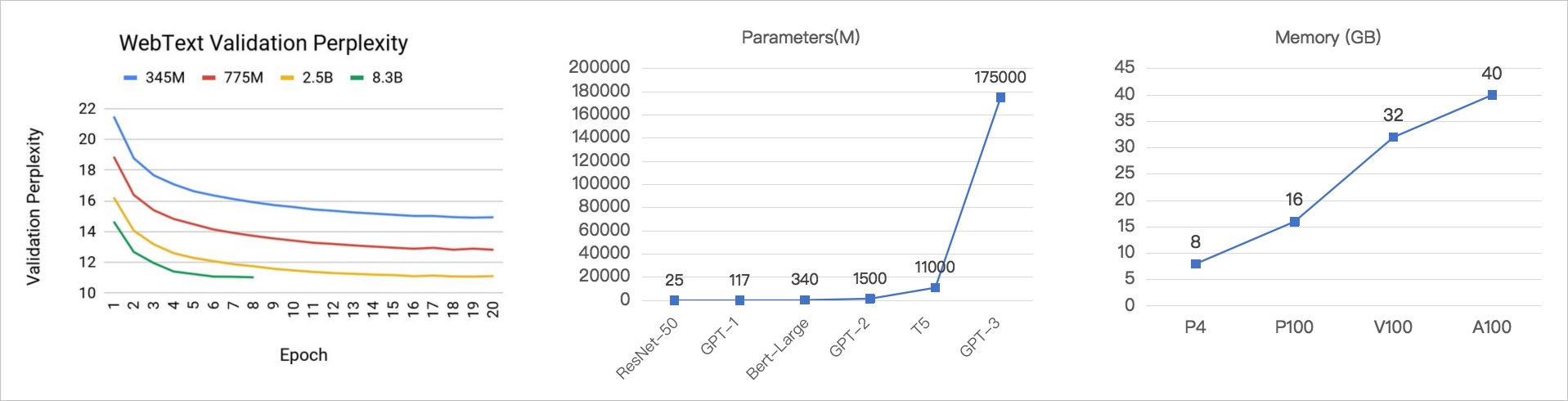The BERT-Large model has achieved good results in many NLP scenarios. The idea of BERT is very close to that of Embeddings from Language Models (ELMo) and GPT. However, BERT supports pre-training on a large unsupervised corpus. The speed of BERT far exceeds that of ELMo. The performance of BERT is better than that of GPT. Therefore, BERT is widely used in real business scenarios.

BERT-Large has only 0.34 billion parameters. Compared with T5 and GPT-3, the parameter scale of BERT-Large is very small. However, the training batch size on the NVIDIA V100 16 GB GPU reaches only 2 to 8. The specific value is related to the embedding size and sequence length. The large scale of model parameters results in a large gradient communication overhead. In addition, the small batch size results in a high communication proportion. Therefore, using traditional data parallelism for training provides extremely poor acceleration results.

## Issues

The following figure shows the structure of the BERT-Large model.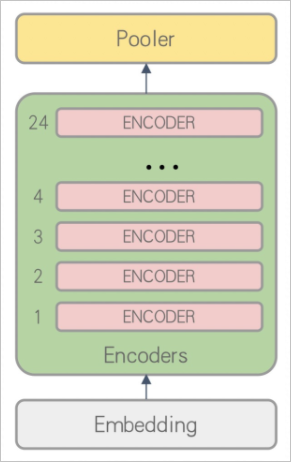The original BERT-Large model uses the following code:
``````embedding_output = embedding(inputs)
encoder_output = encoder_layer_1_24(embedding_output)
pooler_output = pooler(encoder_output)``````
Assume that the value of the max_seq_len parameter is 384. In the data parallelism scenario, the maximum batch size of the model reaches only 6 on a single V100 GPU that has 16 GB of video memory. Each round of iteration synchronizes 1.245 GB of gradients. In a network environment that provides a 50 Gbit/s bandwidth, the communication time is 398.4 ms, which is calculated based on the following formula: `2 × 1.245 GB/50 Gbit/s = 398.4 ms`. The process of reading training data also consumes network bandwidth, so the actual communication time is longer than this value. Large-scale training has the following issues:
• The batch size is too small. As a result, the model greatly fluctuates and the convergence is poor.
• The gradient communication traffic is large, and the small batch size increases the proportion of training communication, resulting in poor distributed scaling.
To resolve the preceding issues, Whale introduces the hybrid parallelism strategy that combines model parallelism, pipeline parallelism, and data parallelism. Whale also supports high-performance communication backend and scheduling optimization to provide you with a high-performance, simple, and easy-to-use distributed training framework. The hybrid parallelism strategy combines the following parallelism strategies:
• Model parallelism
• The model is divided into different stages at the layer granularity and assigned to different GPUs for execution. This reduces the video memory usage of the model on each GPU and increases the batch size.
• Activation communication is used between stages instead of gradient synchronization to greatly reduce the communication traffic between GPUs. For example, in the BERT-Large model, only 27 MB of activation data needs to be transmitted per iteration.
• The batch size is increased to make the training more stable and achieve better convergence.
• Pipeline parallelism

If only model parallelism is used, GPUs depend on each other to run tasks. Therefore, only one GPU can run tasks at a time, while other GPUs are idle. This results in low GPU utilization. The pipeline parallelism strategy designed by Whale allows you to train multiple mirco-batches at a time. Each mini-batch corresponds to a micro-batch based on the strategy. Different GPUs can run different stages of the pipeline at the same time. This improves GPU utilization.

• Data parallelism

The hybrid parallelism strategy that combines model parallelism and pipeline parallelism is limited by the total number of layers in the model and the efficiency of pipeline parallelism. Therefore, the hybrid parallelism strategy does not allow infinite distributed scaling. In scenarios where ultra-large-scale training data is involved, data parallelism is required to perform distributed scaling.

## Solution

The hybrid parallelism strategy that combines model parallelism, pipeline parallelism, and data parallelism is used to improve the distributed performance of the BERT-Large model. This section describes the detailed solution.
1. Model parallelism

The batch size of the BERT-Large model typically reaches only 2 to 8. The specific value is related to the embedding size and sequence length. As a result, the model greatly fluctuates and the convergence is poor. You can divide the model into multiple stages by layer and assign these stages to different GPUs for distributed training. This way, model parallelism is implemented.

Regardless of the bandwidth and hardware topology, model division may be affected by load balancing, including video memory balancing and computing power balancing. The activation, video memory usage, and floating-point operations per second (FLOPS) are almost the same among the layers of the BERT-Large model. For the purpose of balancing video memory usage and computing power among different stages, Encoder layers 1 to 8, Encoder layers 9 to 16, and Encoder layers 17 to 24 of the BERT-Large model are assigned to different GPUs for training. The following figure shows the computing diagram after parallelization.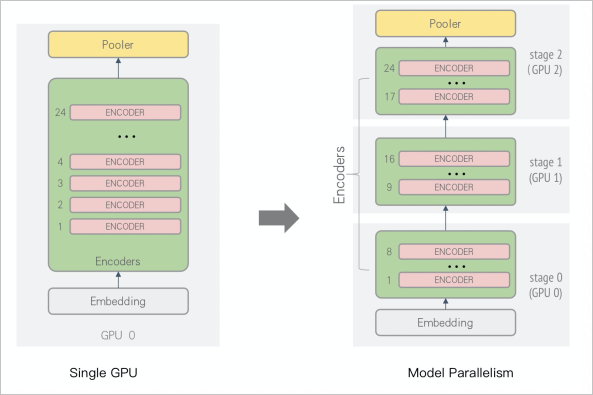The embedding layer and Encoder layers 1 to 8 are assigned to GPU 0 for computing, Encoder layers 9 to 16 are assigned to GPU 1 for computing, and Encoder layer 17 to 24 and the pooler layer are assigned to GPU 2 for computing, as shown in the preceding figure. This method has the following benefits:
• The size of the model assigned to each GPU is greatly reduced, so the batch size can be increased to accelerate convergence.
• Only activation data needs to be transmitted among GPUs. About 27 MB of activation data is transmitted in each iteration round. This eliminates the gradient communication process.
• In the scenario where the model is too large and cannot be stored in the video memory of a single GPU, the layer-based model parallelism strategy makes model training possible.
2. Pipeline parallelism
If only model parallelism is used for distributed training, only one GPU can run tasks at a time, while other GPUs are idle. This results in low GPU utilization, as shown in the following figure.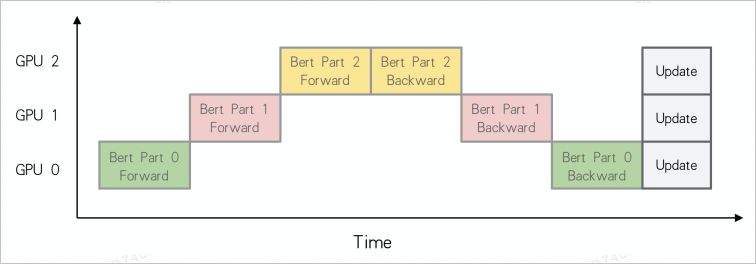In the preceding figure, only one GPU is running the Forward or Backward task at each point in time, while other GPUs are idle and wait. Whale designs the pipeline parallelism strategy to improve GPU utilization in model parallelism scenarios. After a micro-batch is trained, each GPU transmits the activation data to the next GPU and immediately trains the next micro-batch. A pipeline is formed among multiple GPUs based on the preceding method. The following figure shows the execution logic.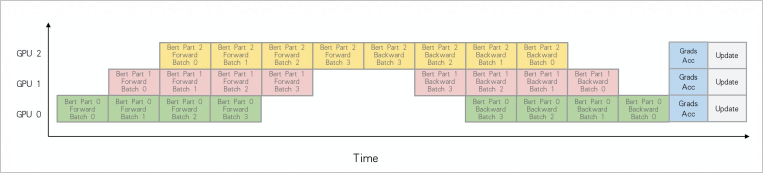In the example shown in the preceding figure, the number of micro-batches is 4. The timeline after the pipeline parallelism optimization shows that multiple GPUs are computing in parallel at the same time. After the four micro-batches are processed, each GPU runs the Grads Acc task to accumulate the local gradients of each micro-batch and updates the weights. Compared with model parallelism, this improves GPU utilization. However, the preceding figure still contains a large number of gaps. This indicates that specific GPUs are still idle at some points in time. In this case, the number of micro-batches for pipeline parallelism can be increased to reduce the idle time. In addition, Whale adopts the Backward-Preferred scheduling policy to adjust the Forward and Backward computing sequence among micro-batches so that the GPUs stay in the computing state as much as possible. This improves the performance of pipeline parallelism and reduces the GPU idle time, as shown in the following figure. In the figure, the number of micro-batches is 5.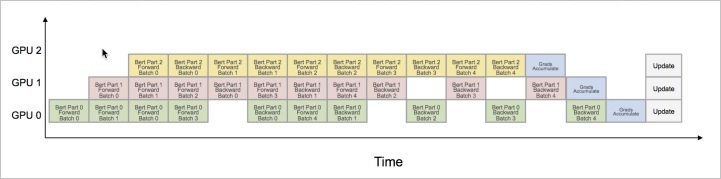3. Hybrid parallelism
The model cannot be divided into infinite stages in model parallelism, and using a large number of stages deteriorates the performance. Therefore, if only model parallelism and pipeline parallelism are used, the performance requirements still cannot be met. The large amounts of training data generated in business scenarios must be processed by large amounts of computing resources in parallel. Therefore, you also need to use data parallelism to resolve the issue of large-scale training data. The following figure shows the computing diagram after data parallelism is used based on pipeline parallelism.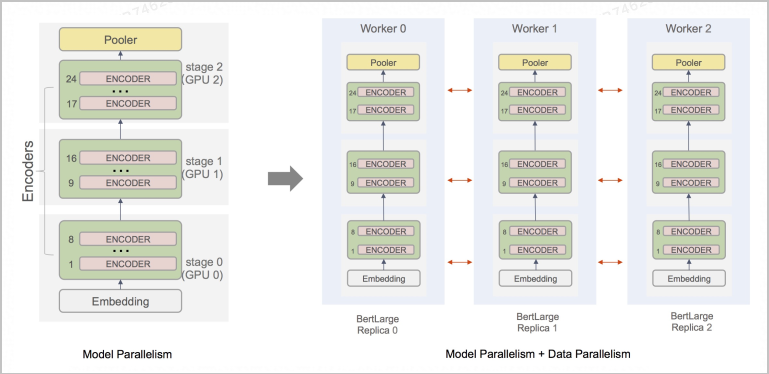In the preceding figure, three workers are used. Each worker has three GPUs. Whale assigns a stage for each worker to the GPUs of the worker to implement pipeline parallelism. After each worker completes training for the micro-batches specified by the batch size, the worker accumulates the local gradients and synchronizes the gradients to other workers by using AllReduce.
Whale performs the following optimization to more efficiently use the inter-GPU NVLink bandwidth on a server or the PCI-e bandwidth if NVLink bandwidth is unavailable, and reduce the overhead of gradient synchronization:
• The model is divided and the stages of the model are assigned to different servers for computing to implement model parallelism.
• Gradient synchronization required by data parallelism is performed inside each server. The communication traffic is about 1.2 GB.
• The activation data that is generated for layer-based model division is transmitted by using TCP. The size of the activation data is about 27 MB.
This method can improve the communication efficiency and reduce the iteration time of each round. To use the optimized parallelism solution of Whale, you do not need to modify the model definition code. You need only to change the value of the layout parameter from column-based to row-based to modify the logic for grouping hardware resources. For more information, see . The following figure shows the computing diagram of the solution.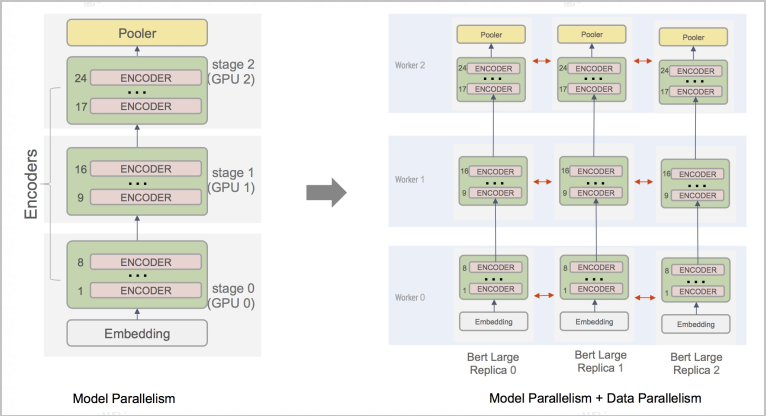## Use Whale to implement pipeline parallelism

The process of implementing pipeline parallelism consists of three steps based on the standard Whale distributed programming paradigm: model division, resource grouping, and mapping.

1. Divide the model.
Specify scopes to divide the model into different stages based on different requirements for video memory and computing power. For more information, see Scope-related operations. In the preceding solution, the model is divided into three stages:
• Stage 0: includes the embedding layer and Encoder layers 1 to 8.
• Stage 1: includes Encoder layers 9 to 16.
• Stage 2: includes Encoder layers 17 to 24 and the pooler layer.
Whale allows you to implement pipeline parallelism by modifying the original code of the BERT-Large model. To do so, perform the following steps:
1. Call `whale.stage` to implement model parallelism. Sample code:
``````import whale as wh

with wh.stage():
embedding_output = embedding(inputs)
encoder_output = encoder_layer_1_8(embedding_output)

with wh.stage():
encoder_output = encoder_layer_9_16(embedding_output)

with wh.stage():
encoder_output = encoder_layer_17_24(embedding_output)
pooler_output = pooler(encoder_output)``````
2. In the code for implementing model parallelism, call `whale.pipeline` to implement pipeline parallelism. Sample code:
``````import whale as wh

with wh.pipeline(num_micro_batch=5): # Process the data of five mini-batches in sequence to implement pipeline parallelism. In each round of iteration, each GPU processes the data of five mini-batches.
with wh.stage():
embedding_output = embedding(inputs)
encoder_output = encoder_layer_1_8(embedding_output)

with wh.stage():
encoder_output = encoder_layer_9_16(embedding_output)

with wh.stage():
encoder_output = encoder_layer_17_24(embedding_output)
pooler_output = pooler(encoder_output)``````
3. In the code for implementing pipeline parallelism, call `whale.replica` to implement data parallelism. Sample code:
``````import whale as wh

with wh.replica():  # Implement data parallelism.
with wh.pipeline(num_micro_batch=5):  # Process the data of a specific number of mini-batches in sequence to implement pipeline parallelism. The number of mini-batches is specified by the num_micro_batch parameter. In each round of iteration, each GPU processes the data of a specific number of mini-batches. The number of mini-batches is specified by the num_micro_batch parameter.
with wh.stage():
embedding_output = embedding(inputs)
encoder_output = encoder_layer_1_8(embedding_output)

with wh.stage():
encoder_output = encoder_layer_9_16(embedding_output)

with wh.stage():
encoder_output = encoder_layer_17_24(embedding_output)
pooler_output = pooler(encoder_output)``````
2. Group resources.
Divide hardware resources into three virtual devices because the model is divided into three stages. Assume that you have applied for three workers, each worker has three GPUs, and the grouping policy is `layout="row"`. For more information, see whale.cluster. The resources are divided into the following three groups:
• Virtual device 0: [[/worker:0/gpu:0], [/worker:0/gpu:1], [/worker:0/gpu:2]]
• Virtual device 1: [[/worker:1/gpu:0], [/worker:1/gpu:1], [/worker:1/gpu:2]]
• Virtual device 2: [[/worker:2/gpu:0], [/worker:2/gpu:1], [/worker:2/gpu:2]]
The Cluster tool provided by Whale allows you to group the applied workers. Sample code:
``cluster = wh.cluster(layout={"row":3})``
3. Map the model to resources.
Map each stage of the model to a virtual device based on the model division and resource grouping results.
• Assign Stage 0 to Virtual device 0 [[/worker:0/gpu:0], [/worker:0/gpu:1], [/worker:0/gpu:2]] based on the data parallelism strategy. Each GPU processes a replica of Stage 0.
• Assign Stage 1 to Virtual device 1 [[/worker:1/gpu:0], [/worker:1/gpu:1], [/worker:1/gpu:2]] based on the data parallelism strategy. Each GPU processes a replica of Stage 1.
• Assign Stage 2 to Virtual device 2 [[/worker:2/gpu:0], [/worker:2/gpu:1], [/worker:2/gpu:2]] based on the data parallelism strategy. Each GPU processes a replica of Stage 2.
Whale allows you to use the `with` syntax on the generated cluster to map the model to hardware resources with ease. The following core code shows how to use Whale to implement parallelism. For more information about the executable code that implements pipeline parallelism, visit pipelined_bert_models_3_staged.py.
``````import whale as wh
cluster = wh.cluster(layout={"row":3}) # Group resources.

with cluster:                         # Map the model to resources.
with wh.replica():
with wh.pipeline(num_micro_batch=5):
with wh.stage():
embedding_output = embedding(inputs)
encoder_output = encoder_layer_1_8(embedding_output)

with wh.stage():
encoder_output = encoder_layer_9_16(embedding_output)

with wh.stage():
encoder_output = encoder_layer_17_24(embedding_output)
pooler_output = pooler(encoder_output)``````

## Performance

The data parallelism performance of Horovod is used as the baseline for comparison. The following table describes the test environment.
Item Operation
GPU model ecs.gn6v-c10g1.20xlarge (V100 × 8)
Network VPC-35 GB
NCCL_MAX_NRINGS The official NVIDIA parameter. The value is set to 4 during the test.
NCCL_MIN_NRINGS The official NVIDIA parameter. The value is set to 4 during the test.
In this topic, the performance of pipeline parallelism is compared with that of data parallelism in the following test scenarios:
• Horovod data parallelism
• Whale data parallelism
• Whale model parallelism + pipeline parallelism
The following figure shows the comparison result.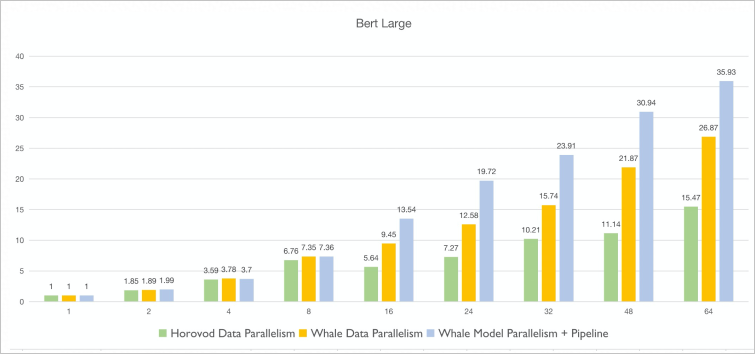Note The X-axis indicates the number of GPUs, whereas the Y-axis indicates the acceleration ratio.
From the preceding figure, you can draw the following conclusions:
• Whale performs better than Horovod in data parallelism scenarios. When 64 GPUs are used, the acceleration ratio of Whale is 1.74 times that of Horovod.
• If the number of GPUs is smaller than 8, the performance of Whale in the data parallelism scenario is not much different from that in the model parallelism + pipeline parallelism scenario. The performance of data parallelism is high because the GPUs inside a server are connected by using high-speed NVLink and the communication efficiency is very high. When the number of GPUs increases and cross-server communication is required, the benefits of pipeline parallelism become apparent.
• When 64 GPUs are used, the throughput performance in the model parallelism + pipeline parallelism scenario is 1.34 times that in the data parallelism scenario.